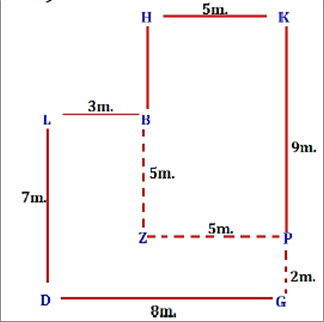sbi clerk :: reasoning :: test 3

Home sbi clerk / reasoning Questions and Answers

1.  Directions(Q.1 to Q.5 ):
In these questions, relationship between different elements is shown in the statements. These statements are followed by two conclusions. Mark answer

Statements:
$A > B \le C = D \le E$
$C \ge F = G > H$
Conclusions:
I. ${\rm{G}} \le {\rm{E}}$
II. ${\rm{A}} > {\rm{H}}$

a

If only conclusion I follows.

b

If only conclusion II follows.

c

If either conclusion I or II follows.

d

If neither conclusion I nor II follows.

e

If both conclusions I and II follow.View AnswerDiscuss
 Answer : Option A Explanation : $G = F \le C = D \le E\left( {TRUE} \right)$ $A > B \le C \ge F = G > H\left( {FALSE} \right)$

2.  Statements:
I.${\rm{H}} \ge {\rm{T}} > {\rm{S}} \le {\rm{Q}}$
II.${\rm{T}} \ge {\rm{U}} = {\rm{V}} > {\rm{B}}$
Conclusions:
I.V > S
II. ${\rm{B}} \le {\rm{H}}$

a

If only conclusion I follows.

b

If only conclusion II follows.

c

If either conclusion I or II follows.

d

If neither conclusion I nor II follows.

e

If both conclusions I and II follow.View AnswerDiscuss
 Answer : Option D Explanation : $V = U \le T > S\left( {FALSE} \right)$ $B < V = U \le T \le H\left( {FALSE} \right)$

3.  Statements:
I.${\rm{F}} < {\rm{K}} \le {\rm{L}}$
II.${\rm{H}} \ge {\rm{R}} > {\rm{K}}$
Conclusions:
I.H > L
II. R > F

a

If only conclusion I follows.

b

If only conclusion II follows.

c

If either conclusion I or II follows.

d

If neither conclusion I nor II follows.

e

If both conclusions I and II follow.View AnswerDiscuss
 Answer : Option B Explanation : $H \ge R > K \le L\left( {FALSE} \right)$ $R > K > F\left( {TRUE} \right)$

4.  Statements:
I.${\rm{N}} \ge {\rm{P}} > {\rm{K}} = {\rm{L}}$
II.${\rm{P}} \le {\rm{Q}} < {\rm{Z}}$
III.${\rm{T}} > {\rm{K}}$
Conclusions:
I. N > Q
II. Z < T

a

If only conclusion I follows.

b

If only conclusion II follows.

c

If either conclusion I or II follows.

d

If neither conclusion I nor II follows.

e

If both conclusions I and II follow.View AnswerDiscuss
 Answer : Option D Explanation : $N \ge P \le Q\left( {FALSE} \right)$ $Z > Q \ge P > K < T\left( {FALSE} \right)$

5.  Statements:
${\rm{P}} < {\rm{H}} = {\rm{O}} \ge {\rm{N}}$
${\rm{E}} \ge {\rm{H}} < {\rm{S}}$
Conclusions:
I.${\rm{N}} \le {\rm{E}}$
II. S > P

a

If only conclusion I follows.

b

If only conclusion II follows.

c

If either conclusion I or II follows.

d

If neither conclusion I nor II follows.

e

If both conclusions I and II follow.View AnswerDiscuss
 Answer : Option E Explanation : $N \le O = H \le E\left( {TRUE} \right)$ ????>????>????(????????????????)

6.  Directions(Q.6 to Q.10 ):
Study the given information carefully to answer the given question.
J, K, L, M, N, O and G are seven different boxes of different colours i.e. Brown, Orange, Silver, Pink, Yellow, White and Green but not necessarily in the same order.
The Box which is of Brown colour is immediately above J. There are only two box between M and the box which is of Brown colour and Box M is above the Brown colour box. The Box which is of Silver colour is above M but not immediately above M. There are only three box between L and the box which is of Silver colour. The box which is of Green colour is immediately above L. The box which is of Pink colour is immediately above the box G. Only one box is there between K and N. Box K is above N. Neither box K nor J is of Yellow colour. J is not of orange colour.

How many box is/are there between M and G?

a

One

b

Two

c

Three

d

Four

e

NoneView AnswerDiscuss
 Answer : Option B Explanation :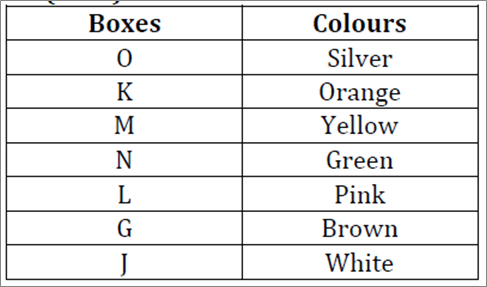7.  What is the colour of ’O’?

a

Green

b

Brown

c

Silver

d

Pink

e

Can’t be determined.View AnswerDiscuss
 Answer : Option C Explanation :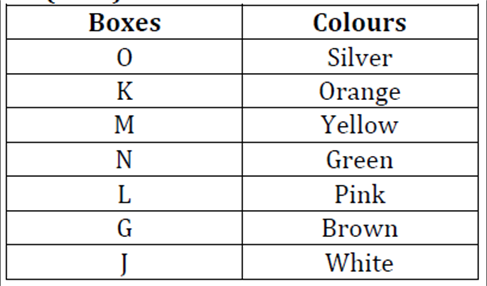8.  Find the pair of colour and boxes which is not correct?

a

K-Pink

b

O-Silver

c

J-white

d

G-Brown

e

None of theseView AnswerDiscuss
 Answer : Option A Explanation :9.  Which of the following condition is correct regarding yellow colour with respect to N?

a

There is one box between N and the Yellow colour box

b

N is immediately above of yellow colour box

c

The Yellow colour box is related to the box which is immediately above N

d

All of the above is true

e

None of the above is true.View AnswerDiscuss
 Answer : Option C Explanation :10.  Which of the following colour belongs to ’J’?

a

Brown

b

Pink

c

Yellow

d

Orange

e

None of these.View AnswerDiscuss
 Answer : Option E Explanation :11.  Directions(Q.11 to Q.13 ):
Study the following information and answer the given question.
• T is the sister of D. D is married to P. P is the son of M.
• T is the mother of J. Y is the father of U.
• Y has only one son and only one daughter.
• U is the daughter of T. Q is the son of D.

How is P related to T ?

a

Brother

b

cannot be determined

c

Brother-in-law

d

Cousin brother

e

UncleView AnswerDiscuss
 Answer : Option C Explanation :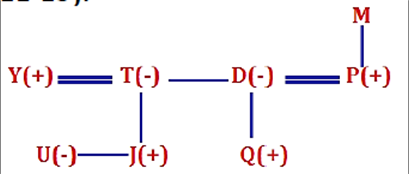12.  How is J related to D ?

a

Son

b

Niece

c

Son-in-law

d

Nephew

e

DaughterView AnswerDiscuss
 Answer : Option D Explanation :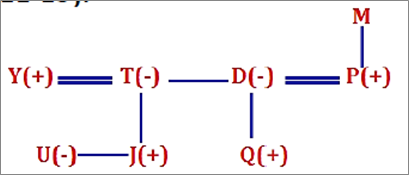13.  If M is wife of W then how is Q related to W ?

a

Son-in-law

b

Grandson

c

Nephew

d

Son

e

Cannot be determinedView AnswerDiscuss
 Answer : Option B Explanation :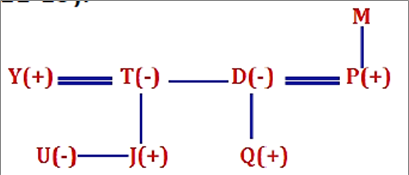14.  Directions(Q.14 to Q.15 ):
P is 9 m to the south of K. K is 5 m to the east of H. H is 4 m to the north of B. L is 3 m west of B. D is 7 m south of L. G is 8 m east of D.

If Point Z is 5 m to the west of point P, then what is the distance between B and Z?

a

8 m

b

9 m

c

5 m

d

2 m

e

6 mView AnswerDiscuss
 Answer : Option C Explanation :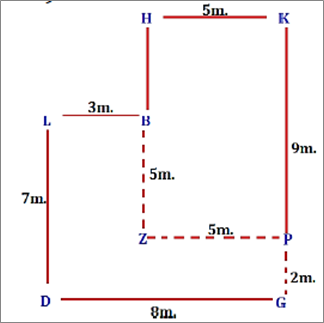15.  How far and in which direction is point K from Point G?

a

11 m to the south

b

7 m to the north

c

11 m to the north

d

7 m to the south

e

11 m to the westView AnswerDiscuss
 Answer : Option C Explanation :